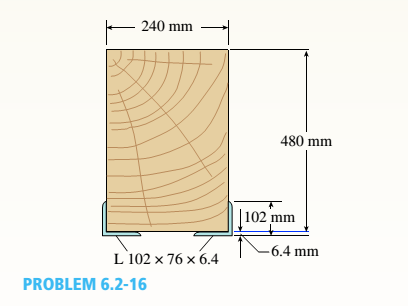# A wood beam in a historic theater is reinforced with two angle sections at the outside lower corners (see figure). If the allowable stress in the wood is 12 M Pa and that in the steel is 140 M Pa, what is ratio of the maximum permissible moments for the beam before and after reinforcement with the angle sections? See Appendix F Table F-5(b) for angle section properties. Assume that e w = 12 GPa and E 3 =210 GPa.### Mechanics of Materials (MindTap Co...

9th Edition
Barry J. Goodno + 1 other
Publisher: Cengage Learning
ISBN: 9781337093347

#### Solutions

Chapter
Section### Mechanics of Materials (MindTap Co...

9th Edition
Barry J. Goodno + 1 other
Publisher: Cengage Learning
ISBN: 9781337093347
Chapter 6, Problem 6.2.16P
Textbook Problem
1 views

## A wood beam in a historic theater is reinforced with two angle sections at the outside lower corners (see figure). If the allowable stress in the wood is 12 M Pa and that in the steel is 140 M Pa, what is ratio of the maximum permissible moments for the beam before and after reinforcement with the angle sections? See Appendix F Table F-5(b) for angle section properties. Assume that ew= 12 GPa and E3=210 GPa.To determine

The ratio of maximum permissible moment of a beam before and after reinforcement.

### Explanation of Solution

Given: .

Area,AL=1090mm2.

Moment of Inertia,I11=1.14×106mm4..

Distance from the neutral axis, d=31mm..

Es =12GPa..

Ew =21GPa..

Width, b=240mm..

Height, h=480mm..

Calculation: .

The maximum moment if no angle section used is calculated as:.

M1max=σwa(bh26).

σwa - Allowable stress..

b- The width of section..

h- Height of section..

M1max=12×(240×48026)M1max=110.592×106N.mm×(1KN1000N)×(1m1000mm)M1max=110.592kN.m.

The location of the neutral axis from the lower end is stated as:.

h2=Ew(bh)(h2+6.4)+Es(2AL)dEw(bh)+Es(2AL)..

Young modulli of steel and wood areEw andEs.

SubstituteEs =12GPa,Ew =21GPa, b=240mm, d=31,Al =1090mm, h=480mm..

h2={12×103(240×480)(4802+604)+{210×103(2×1090)31}{12×103(240×480)}+{210×103(2×1090)}}.

h2={3.4062×1011}+{0.141918×1011}{13.824×108}+{4.578×108}.

h2=0.192813×103mmh2=192.813mm.

The distance of the neural axis from the top..

h1=h+6.4h2.

Substitute h=480mm andh2 =192.813mm.

h1=480+6.4192.813h1=293.587mm.

Calculate the moment of Inertia for wood section..

Iw=bh312+(bh)(h2h1)2.

Substitute b=240mm, h=480mm andh1 =293.587mm.

Iw=240×480312+(240×480)(4802293.587)2.

Iw=22.1184×108+3.3080×108Iw=25.43×108mm4×(1m41000mm4)Iw=2.543×103m4.

The moment of Inertia of the steel section..

Is=2I11+2AL(h2d)2.

SubstituteI11 =1.14×106mm4 ,h2 =192.813mm,AL =1090mm , d=31mm..

Is=2(1.14×106mm4)+2(1090)(192.81331)2.

Is=0.0228×108+0.570799×108Is=0.5936×108mm4×(1m410004mm4)

### Still sussing out bartleby?

Check out a sample textbook solution.

See a sample solution

#### The Solution to Your Study Problems

Bartleby provides explanations to thousands of textbook problems written by our experts, many with advanced degrees!

Get Started

Find more solutions based on key concepts
What are the major differences between aerobic and anaerobic sealants?

Automotive Technology: A Systems Approach (MindTap Course List)

What is a table, and what role does it play in the relational model?

Database Systems: Design, Implementation, & Management

Explain what is meant by object-oriented syntax.

Engineering Fundamentals: An Introduction to Engineering (MindTap Course List)

How would you balance DFDs?

Systems Analysis and Design (Shelly Cashman Series) (MindTap Course List)

Explain the issues surrounding companies using customer data.

Enhanced Discovering Computers 2017 (Shelly Cashman Series) (MindTap Course List)

What characteristics of the weld bead do the weaving of the electrode cause?

Welding: Principles and Applications (MindTap Course List)

If your motherboard supports ECC DDR3 memory, can you substitute non-ECC DDR3 memory?

A+ Guide to Hardware (Standalone Book) (MindTap Course List)